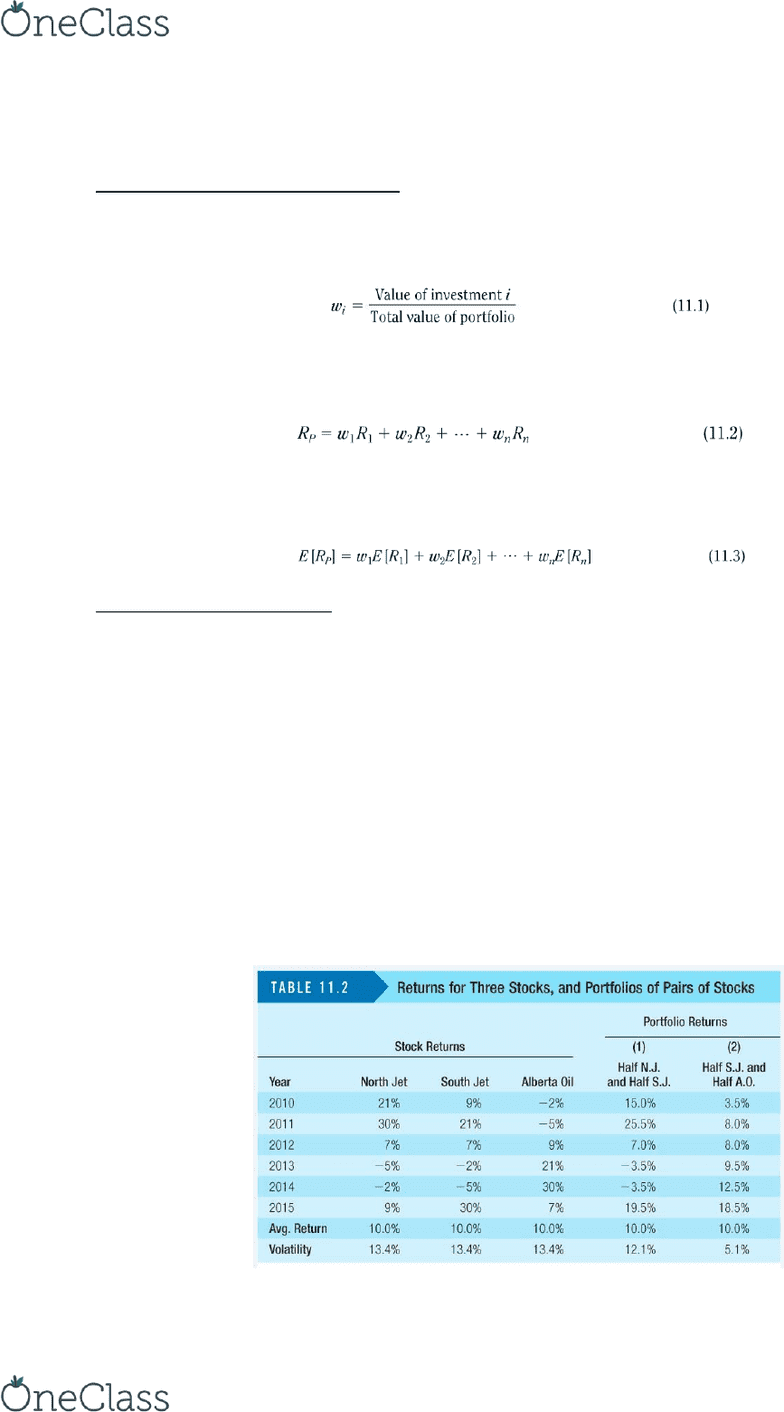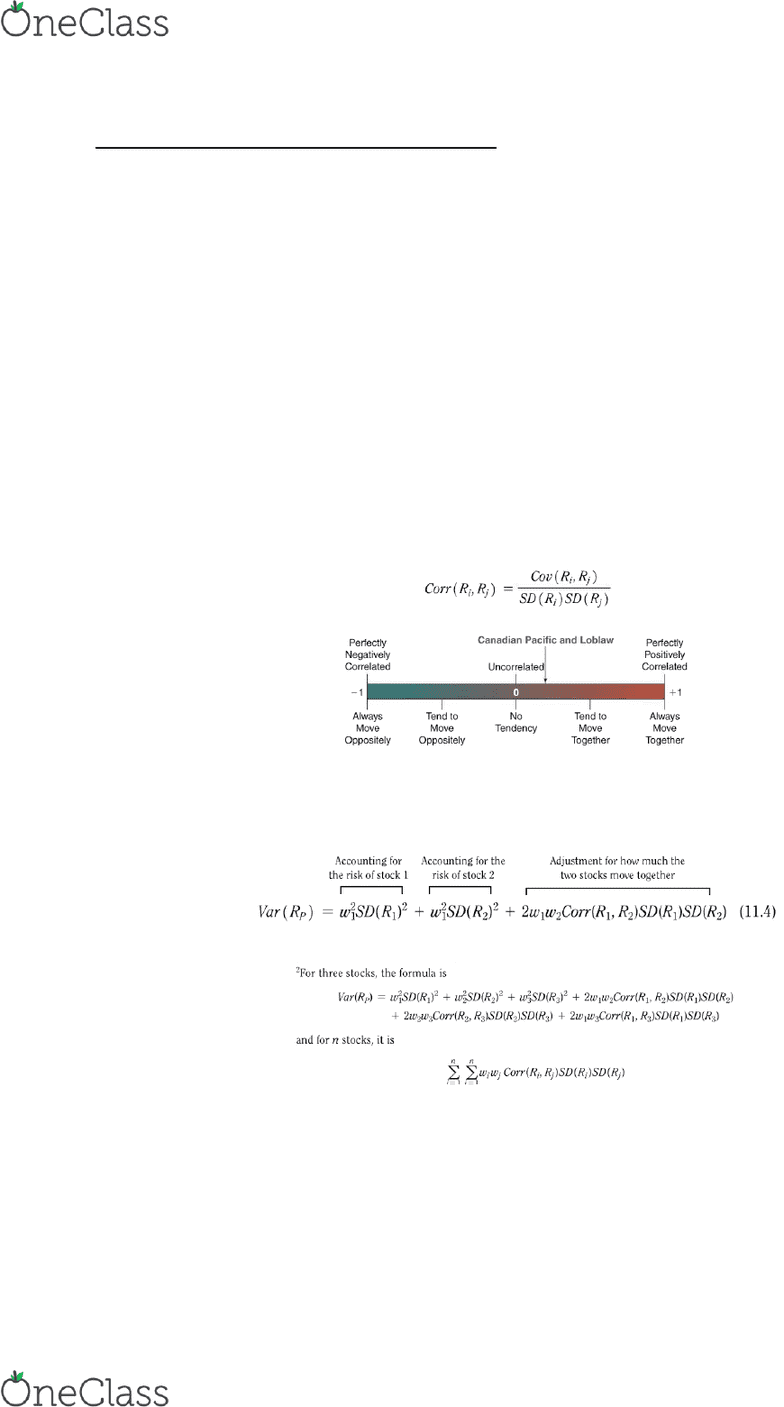Textbook Notes (280,000)
CA (170,000)
UOIT (400)
BUSI (200)
Chapter 11

# BUSI 2402U Chapter Notes - Chapter 11: Risk Premium, Westjet, Weighted Arithmetic Mean

School
UOIT
Department
Course Code
BUSI 2402U
Professor
Dr.K.Smimou
Chapter
11

This preview shows pages 1-2. to view the full 6 pages of the document.CHAPTER 11: Systematic Risk and the Equity Risk Premium
The Expected Return of a Portfolio
Portfolio Weight the fraction of the total investment of each stock (WestJet, Hasbro,
Nike, etc.) in a portfolio.
Return on a Portfolio the weighted average of the returns on the investments in the
portfolio, where the weighs correspond to portfolio weights.
Expected Return of a Portfolio the weighted average of the expected returns of the
investments within it, using the portfolio weights.
The Volatility of a Portfolio
Volatility of a Portfolio the total risk, measured as SD, of a portfolio.
Diversifying Risks
Investors care about return, but also risk.
By combing stocks into a portfolio, we reduce risk through diversification.
The amount of risk that is eliminated in a portfolio depends on the degree to which the
stocks face common risks and move together. For example, because the two airline
stocks tend to perform well or poorly at the same time, the portfolio of airline stocks
has a volatility that is only slightly lower than the volatility of the individual stocks. The
airline and oil stocks, on the other hand, do not move together almost in opposite
directions. As a results, more risk is cancelled out, making that portfolio less risky.
find more resources at oneclass.com
find more resources at oneclass.com

Only pages 1-2 are available for preview. Some parts have been intentionally blurred.Measuig Stoks’ Co-Movement: Correlation
Correlation a measure of the degree (ranging from -1 to +1) to which returns share
common risk, calculated as the covariance of the returns divided by the SD of each
return.
The closer the correlation is to +1, the more the returns tend to move together as a
result of common risk.
When the correlation equals 0, the returns are uncorrelated they have no tendency to
move together or in the opposite directions.
The closer the correlation is to -1, the more the returns tend to move in opposite
directions.
Stock returns will tend to move together if they are affected similarly by economic
events. So, stocks in the same industry tend to have highly correlated returns than
stocks in different industries.
See page 381 to learn how to calculate correlation on Excel!
Copaig a Potfolio’s Vaiae ad SD
The formula for the variance of a two-stok potfolio is…
With a positive amount invested in each stock, the more the stocks move together and
the higher their correlation, the more volatile the portfolio will be. The portfolio will
have the greatest variance if the stocks have a perfect positive correlation (+1).
The expected return of a portfolio is equal to the weighted average expected return of
its stocks, but the volatility of a portfolio is less than the weighted average volatility. As
a result, its clear that we can eliminated some volatility by diversifying.
find more resources at oneclass.com
find more resources at oneclass.com